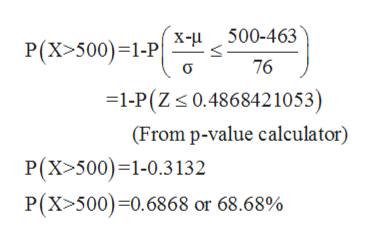# The Rudolph has a mean flying speed of 463 mph, with a standard deviation of 76 mph. These statistical values were established over the past 50 years. Answer the following questions:What proportion of Rudolph's flying speeds is above 500 mph?

Question
9 views

The Rudolph has a mean flying speed of 463 mph, with a standard deviation of 76 mph. These statistical values were established over the past 50 years. Answer the following questions:

What proportion of Rudolph's flying speeds is above 500 mph?

check_circle

Step 1

From the given information, mean (μ) =463 mph and standard deviation (σ) =76 mph

Let ‘X’ be a normal distribution random variable.

Step 2

Let’s find,

Proportion of Rudolph’...help_outlineImage TranscriptioncloseX-500-463 P(X>500) 1-P 76 =1-P(Z 0.4868421053) (From p-value calculator) P(X>500) 1-0.3132 P(X>500) 0.6868 or 68.68% fullscreen

### Want to see the full answer?

See Solution

#### Want to see this answer and more?

Solutions are written by subject experts who are available 24/7. Questions are typically answered within 1 hour.*

See Solution
*Response times may vary by subject and question.
Tagged in

### Statistics